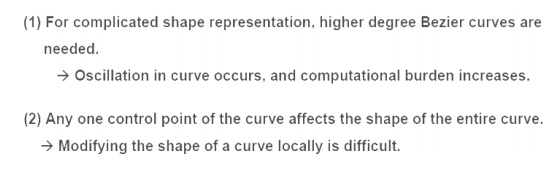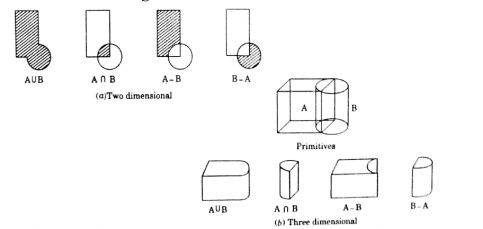Home | | Computer Aided Design and Manufacturing | Important Questions and Answers: Geometric Modeling

# Important Questions and Answers: Geometric Modeling

Mechanical - Computer Aided Design - Geometric Modeling

GEOMETRIC MODELING

1.     What are the two types of equations for curve representation?

(1) Parametric equation x, y, z coordinates are related by a parametric variable (u or θ)

(2) Nonparametric equation x, y, z coordinates are related by a function

Example: Circle (2-D)Name some types of curves used in geometric modelling.

Hermite curves

Bezeir curves

B-spline curv es

NURBS curv es

3   What are the desirable properties of Bezier Curve?4 Write any Two Drawbacks of Bezier Curves.5.  List the advantages of B -spline curves.6.  What are the functions of Geometric Modelling in design analysis?

Evaluation of are a, volume, mass and inertia properties Interference chec king in assemblies

Analysis of tolerance build-up in assemblies Kinematic analys is of mechanisms and robots Automatic mesh generation for finite element analysis

7.  What are the functions of Geometric Modelling in Manufacturing??

Parts classification Process planning

NC data generatio n and verification Robot program ge neration Scheduling

8.     List the Properties of a Geometric Modeling System.

The geometric model must stay invariant with regard to its location and o rientation The solid must have an in terior and must not have isolated parts

The solid must be finite a nd occupy only a finite shape

The application of a trans formation or Boolean operation must produce another solid The solid must have a finite number of surfaces which can be described

The boundary of the solid must not be ambiguous

9.  What are called 2 ½ - D Wire frame models?

Two classes of shape for which a simple wire-frame representation is often adequate are those shapes defined by projecting a plane profile along its normal or by rotating a planar profile about an axis. Such shapes are not two-dimensional, but neither do they require sophisticated three-dimensional schemes for their representation. Such representation is called 2 ½ - D.

10. Draw an example for 2 ½ - D Wire frame model.11.                        Catalog Techniques In Surface Modelling.

i.           Surface Patch

ii.           Coons Patch

iii.           Bicubic Patch

iv.          Be’zier Surface

v.           B-Spline Surfaces

12.                        What are the Solid Modeling Techniques?

The various methods for representing the solids are:

1.     Half-space method

2.     Boundary representation method (B-rep)

3.     Constructive solid geometry (CSG and C-rep)

4.     Sweep representation

5.     Analytical solid modeling (ASM)

6.     Primitive instancing

7.     Spatial partitioning representation

a.     Cell decomposition

b.     Spatial occupancy enumeration

c.      Octree encoding

13.                        Write short note on Be’zier Surface.

The Be’zier s urface formulation use a characteristic polygonPoints the Be zier surface are given by14.                        Write any topological te rms used Boundary representation method (B-rep).

Vertex (V) : It is a unique point (an ordered triplet) in space

Edge (E): It is finite, non-self intersecting, directed space c urve bounded by t wo vertices that are not necessarily distinct

Face (F) : It is defined as a finite connected, non-self-intersecting, region of a closed oriented surface bounded by one or mor e loops

Loop (L) : It is an ordered alternating sequence of vertices and edges

Genus (G) : It is the topological name for the number of handles or through holes in an object

Body/Shell(B) : It is a set of faces that bound a single connected c losed volume. A m inimum body is a point

15. What is called singular body in b-rep?

A minimum body is a point; topologically this body has one face, one vertex, and no edges. It is called a seminal or singular body

16.                        Sketch some open polyhedral objects used in B-rep.17. Write Euler’s formula for open and closed objects used in b-rep.

Euler – Poincare Law for closed objects  :

F – E + V – L = 2 (B – G)

Euler – Poincare Law for open objects   :

F – E + V – L =  B – G

Appropriate to construct solid models of unusual shapes

Relatively simple to convert a b-rep model to wireframe model

Requires more storage

Not suitable for applications like tool path generation

O   Slow manipulation

19.            How solids are created using CSG?20. Give an example for CS G Tree.Study Material, Lecturing Notes, Assignment, Reference, Wiki description explanation, brief detail
Mechanical : Computer Aided Design : Geometric Modeling : Important Questions and Answers: Geometric Modeling |